## General Equation for Electromagnetic Relay:

It has already been shown that when not more than two quantities are involved, the equation for the characteristic of the relay at the threshold of operation under steady state conditions, when plotted on complex planes is a circle. The General Equation for Electromagnetic Relay can be represented in a general form as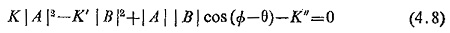where

|A| and |B| – two quantities being compared

Φ – electrical angle between A and B

θ – relay characteristic angle, which is the value of Φ for maximum torque

K and K’ – scalar constants

K – mechanical restraining torque

This Eq. (4.8) is similar to Eqs. (4.4) and (4.6) in nature and is applicable to most of the common types of relays. If the two input quantities are current I and voltage V then the equation for threshold operation becomes: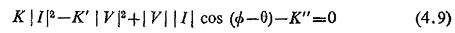which can also be explained as: the current winding produces a torque KI2 and the potential winding a torque K’V2, while the torque due to interaction of current and potential windings will be VI cos (Φ-θ).

K” is finite only in singe quantity relays, where it is used as a level indicator; it is made negligibly small in relays with more than one input so K” = 0 and dividing throughout by K′ |I|2 Eq. (4.9) reduces to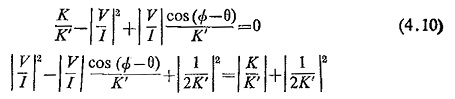Equation (4.10) is similar to Eqs. (4.5) and (4 7).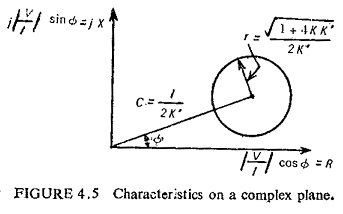This represents a circle on a complex plane, having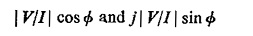as coordinates, i.e. on the R-X plane (Fig. (4.5)).

Scroll to Top